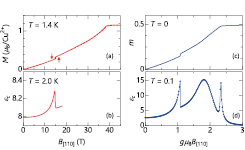Home　>　 About ISSP　>　 Publications　>　Activity Report 2018　>　Xxxx Group

## Magnetoelectric Behavior from Square Cupola Magnetic Units in High Magnetic Field

A particular class of magnetic order with broken space-inversion and time-reversal symmetries has recently attracted considerable interest because it can exhibit symmetry-dependent unique phenomena, such as linear magnetoelectric (ME) effects and associated nonreciprocal optical responses. In exploring such a magnetic order, a material composed of inversion asymmetric magnetic units is fascinating, because structural asymmetry of the unit may stabilize a nontrivial non-coplanar spin arrangement due to asymmetric Dzyaloshinskii-Moriya (DM) interactions, which would lead to various magnetoelectric responses. Recently, we have synthesized new antiferromagnetic insulators A(TiO)Cu4(PO4)4 (A = Ba, Sr, and Pb), which consists of Cu4O12 convex-shaped magnetic units known as square cupola [Inset of Fig. 1(a)] [1-4]. Their crystal structure belongs to a tetragonal space group P4212 and is characterized by a layered arrangement of Cu4O12 square cupolas. Interestingly, neutron diffraction study  reveals that below the antiferromagnetic ordering temperature TN, four Cu2+ spins of each square cupola forms a magnetic quadrupole moment, which, along with magnetic monopole and toroidal moments, is known as one of cluster multipole moments that satisfy the symmetry condition for the linear ME effect. Indeed, we observed a magnetic-field-induced electric polarization in the Pb system and a sharp dielectric anomaly at TN in the Ba and Sr systems. (The difference originates from a stacking manner of magnetic layers: the quadrupole moments stack ferroically in the Pb system, while antiferroically in the Ba and Sr systems.) These results demonstrate that magnetic square cupolas are promising ME-active structural units in the low magnetic field (B) quadrupole phase. However, their ME activity in a B-induced phase, which appears commonly in these materials above 10 T, has not been elucidated. In this study, we have investigated ME properties in the B-induced phases of the Pb  and Sr  systems.Fig. 2. Magnetic field dependence of magnetization (M) and dielectric constant along the c axis (εc) obtained in (a) and (b) experiments and (c) and (d) theoretical calculations for Sr(TiO)Cu4(PO4)4 (see Ref.  for details). The magnetic field was applied along the  axis.Fig. 1. Magnetic field dependence of magnetization (M) and electric polarization along the c axis (Pc) obtained in (a) and (b) experiments and (c) and (d) theoretical calculations for Pb(TiO)Cu4(PO4)4 (see Ref.  for details). The magnetic field was applied along the  axis. The inset of (a) shows a Cu4O12 square cupola magnetic unit.

Figures 1(a) and 1(b) show a B-dependence of electric polarization along the c axis (Pc) in the Pb system, together with magnetization along the  axis (M) for comparison. The B along the  direction (B) was applied with use of a pulsed magnet. It is observed that Pc develops upon the onset of the B-induced phase at 16.4 T. A clear PE hysteresis loop was observed at steady B of 18 T, confirming the ferroelectric nature. Moreover, P shows a B-induced sign reversal around 35 T. On the basis of the space group P4212 in the paramagnetic phase and the existence of finite M and Pc, a possible maximal magnetic point group of the B-induced phase is 2´, which supports a cluster multipole moment composed of toroidal and magnetic moments. Contrary to the Pb case, no finite Pc was observed in the B-induced phase of the Sr system. Instead, as shown in Figs. 2(a) and 2(b), a sharp peak in the dielectric constant along the  axis (εc) appears at the transition field of ~15.0 T. (εc was measured up to 18 T using a superconducting magnet.) Considering the antiferroic stacking of magnetic layers at a low B quadrupole phase, the B-induced phase of the Sr system is expected to be antiferroelectric with a staggered electric polarization along the c axis.

To understand the origin for the experimental results, we have constructed a spin-1/2 effective model, in which a DM interaction between neighboring spins due to convex geometry is taken into account. As shown in Figs. 1(c) and 2(c), this model well reproduces the experimental M curves in both systems, which strongly supports the validity of our model analysis. Next, we evaluated the B dependence of Pc in the Pb system and εc in the Sr system on the basis of the so-called exchange striction mechanism. As shown in Figs. 1(c) and 2(c), the calculated Pc and εc are qualitatively in good agreement with experimental results. The analysis thus indicates that the exchange striction plays a prime role for the ME behavior from Cu4O12 square cupolas in the B-induced phase. Moreover, a cluster multipole decomposition was applied to the calculated spin arrangement of square cupolas. The result shows that both toroidal and quadrupole moments become finite in each magnetic layer. This can explain the ferroelectricity and antiferroelectricity along the c axis induced by B.

The present results thus demonstrate that Cu4O12 square cupolas are promising ME active structural units in a broad range of a magnetic field. Distinct types of ME-active multipole moments can appear in different phases. The convex shaped geometry of Cu4O12 square cupolas plays an important role for the onset of such ME active multipole moments. This implies that not only the square cupola unit, but also other types of convex-shaped structural units are worth to be explored for new magnetoelectric materials.

##### Authors
• K. Kimuraa, Y. Katob, K. Yamauchic, A. Miyake, M. Tokunaga, A. Matsuo, K. Kindo, M. Akaki, M. Hagiwara, S. Kimurad, M. Toyodae, Y. Motomeb, and T. Kimuraa